main page   list of papers   library

THE ORIGIN OF MASS

by Means of the Natural Aether Disturbance

Dedicated to bright memory of my daughter Anastasia

(abbreviated Internet version)

This paper show, that the inertia is exclusive property of Aether reflecting process of power dynamic interaction of the substance and the field with Aether. It is shown, that except for the gravitational mass, as measure of a hydraulic disturbance in Aether, and for the rest mass, as measure of inertness of substance in Aether, the remaining mass measures in modern physics are artefacts. The new concepts of substantional and reactive inertia are determined, which are simple invariant measures of inertness and energy changes for Aether, field and substance.

Taking as the fact  Aether existence in the Universe - unified quasiisotropic, practically incompressible and ideal elastic medium being initial matter - carrier of all energy, all processes happening in the Universe, and taking for the basis of notions about it the working model, developed by present author [2-9], presenting it as bicomponent domain medium - corpuscular and phase, we will consider concept of inertia and problems bound with origining of physical masses in Aether.

Centenary Hoax

The twentieth century was enveloped by relativistic idea of a mathematically selfcontained world, militant idea, not only negating all, that is not entered in its scholastic frameworks, but also carefully deleting all, contradicting to it. It is necessary to recollect the odious Order of Presidium of Academy of Sciences of the USSR, 1964 about interdiction of relativity theory critics.

Through eliminating of physical dimensions, relativists gelded physical sense of the quantitative formulas, having changed the physically interpreted value of phenomena by logically contradicted concepts, having made the theory of physics indeed independent from physics itself.

Massless Physical Measures

As it became clear even within the framework of a relativistic physics, in the nature there are massless forms of the matter, such as an electromagnetic field. However to all kinds of matter the quantity of mass equivalent of its energetic contents was put. Is it correct? Let's show, that it is not.

Outgoing from the study, conducted by the author, and the model of Aether [2-9], developed by him it follows, that the Aether initially has no property of mass. Let's show, what it is not required. Really, outgoing only from classic concepts of physical force, spaces and time, it is possible to derivate the basic physical concepts both measures for Aether and field inside it, not addressing to concept of mass.

The world exists in the time, but not together with the time"

St. Augustin

Concept of the Time. All phenomena in the world, the world by itself exists in the time, as continuous unidimensional sequence of its events. The time, being the basis of the causality can not be rigged: shrink or spread. It would be contradicted to logics and would make wanton logical constructions. The Newtonian time, which is defined still by philosophers of antiquity, is the main reference system, in which one there are all natural phenomena. Even if the subjective time of any object, system seems changeable, it can sees only within the framework of its interaction with other objects having diverse pace of events, that at all does not put under doubt absoluteness and uniform of common time of the world.

" I do not make hypotheses"

I. Newton

Concept of the Space. Rejection of three-dimensional space of the Universe till now is substantial not discovered, but it is only hypothetical constructions, which is possible to refuse for more prosaic models. And, as the science pursues ordering and simplification of a composite picture of the world for its adequate comprehension and usage in needs of the people, the artificial complicating of model of the space only withdraws us from the goal.

Linear Measures. From antique times in physics one (length, distance), two (square, areas) and three (volumes) of spatial dimensions within the framework of three-dimensional space of Euclid are used. As well as it is accepted we will use dimensions l [m], s [m2], V [m3]. The linear measures in physics and in theory of Aether in particular, allow us to use spatial measures for definition of location of physical objects, their value and other quantitative measures of the form.

Angular measures. The dimension of the angle often is skipped in the formula. Therefore the physical sense of value either is lost or is distorted. For example at usage of value of radius, which one often is not a just linear measure, but geometrical place of points of attitude (relation) of a linear measure to angular, that is substantial by a spiral of Archimedes - r [m/radian].

Time-space measures. Together with spatial measures the time allows us to evaluate dynamics of physical objects. Two measures will usually be used: first and second derivatives on time - velocity v [m/s] and acceleration a [m/s2].

Derivatives on time from an angular measure - angular rate ω [radian/s] and angular acceleration ε [radian/s2] concern to time-space measures also.

Force. This concept can be entered irrespective of concept of mass, though the modern theories of physical dimensions will use for the description of structure of dimension of force the measure of mass, as for example [N] = [kg m / s2].

As in physics of 20-th century there is a set of not docked concepts of mass (rest mass, gravitational, inert, longitudinal, transversal and relativistic mass), such definition in any way impossible to call unambiguous. Let's show, that for a nonsubstantial matter, that is for Aether and field it is possible to do without concept of mass, using only concept of force, and then derivative measures of it. Let's begin from a concrete example. The Aether within the framework of model, offered by author, represents an infinite (ecumenical) massif of practically incompressible identical and massless units - amers. This massif is in a condition of comprehensive contraction under pressure 

p = F/S = 2.12·10 81 [N/m2]

In a mechanics it is easy to imagine "light" massless volume, fixed by indirect force, the value of which one in any way is not connected neither with "weight" of this volume, nor with "mass" of "tool", compressing this volume. For example, for true (ideal) gas the pressure is not a function of mass of particles, but only of force of its contraction. Similar measures are the modulus of elasticity, which one also are determined by relation of force to the area or to product of linear parameters of a place of application of the force.

The impulse of force, momentum is product of force on time of its application, and in case, when the force is not a constant - integral on time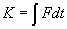Such mass undependent expression of a momentum will be used in all applications, where the value of mass is variable or undefined, for example in astronautics.

Activity. As the natural outcome of operation of force in space will be used a measure of activity, which one determines energy transferred to object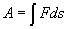The force function is more general measure permitting to evaluate as activity, and other power processes and condition. It can be expressed by the way of differential 

dU = Fxdx + Fydy + Fzdz [N m], [J]

And force to express through a potential force function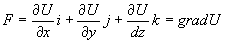And, in this case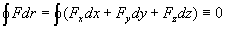Moment of force concerning a point O. For this physical measure expressing a vector product of radius - vector ri, conducted from a point O exactly applications of the force, on force vector Fi 

The moment of momentum can be expressed by two means. As product of a momentum on radius vector 

Or as product of the moment on time of its appendix

Concept of inertia

Let's show, that the concept of inertia is more steep and general, rather than concept of mass. It is related to concept of energy. The phenomenon of inertia is a direct consequence of the law of conservation of momentum and energy.

Measure of inertia of object is identifiable in a general view as relation of force applied on it, referred to acceleration, called by this force, or as increment of a momentum to incremental velocity

in = F/a = Fdt/dv [Ns2/m]

This definition is formally equivalent to concept of mass, though any mass actually here no.

In the same way it is possible to define and differential measure of inertia as its(her) density, that is value come per unit volume V

ρ = d(in) /dV [Ns2/m4]

The given definition is formally equivalent mass densities, however here too there is any mass.

These expressions can be applied for pure massless processes, such as Aether and field. Earlier author in  entered concept of dynamic density of Aether, which one has this sense and determines its inertial properties.

Reasons of origin of inertia

According to the developed working model the Aether represents perfect fluid, for which has the property of superfluidity. It means, that for bodies moving in Aether uniformly and rectilinear, it does not create mechanical resistance.

However at accelerated motion there is a resistance force, appropriate to this acceleration. This force also is inertia.

The corpuscular Aether represents by identical massless gyroscopic units, which one can be presented by the way of identical balance gyros with an arm of force, equal two amer radii, moment of momentum, equal Planck's constants. The rotation axes of these gyros are oriented by a random image, so that in usual condition Aether is the massif of gyros has no any anisotropy.

At uniform motion of body in Aether property of gyroscopic forces and gyroscopic properties of Aether determine the zero resistance of motion.

As is known, for gyroscopic forces the following equation is true 

Fi dri = 0 or ∑ Fi vi = 0 (1)

Where ri – are radii-vectors of force application points;

vi – is a speed of these points.

That is sum of their activities (powers) at any movement of a system, on which one these forces act, is peer to null.

Known particular cases of gyroscopic forces are force of Coriolis

FCor = -2 m v]

where m – is a mass of object;
ω – is an angular rate;
v – is a peripheral speed.

And magnetic component of force of Lorentz

FLor = e [v B] /c

Where e – is electric charge,

c - a speed of the light,

B – is a magnetic induction.

However at accelerated motion there is a reconfigurating of Aether around of a body, that is change of a kinetic energy of amer-gyros. It upsets condition (1), as (1) take place only at summation on selfcontained segments of path or then, when an end position equivalently initials in power sense.

Thus there is a reallocation of energy between is accelerated by a driving body and Aether, that is accumulation of energy in Aether or to the contrary - return of energy of Aether to a body.

This process of exchange of energy happening timelagged, dependent from quantity of matter, also is inertia. Such inertia we shall call substantional (ins).

During radiation of a wave of a field in Aether there is diverse type of inertia - reactive inertia (inr). It arises for the same reason, as the substantional inertia, however that a wave has no property of an elasticity of form of a rigid body, the reactive inertia is reallocation of energy between a local potential energy of Aether and his(its) local kinetic energy. The speed of wave propagation in a phase Aether is determined by speed last, and it is peer to known speed of light.

Reactive inertia. This measure, quantitative value of which was discovered by S. T. Preston in 1875 , and then is described be Henri Poincare in 1900  and Olinto de Pretto (1904), reflects relation between inertia and electromagnetic energy.

For reactive inertia it is possible to write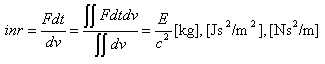The specific reactive inertia come per unit volume of Aether was retrieved by present author in  as a dynamic density of Aether.

Substantional inertia. This measure practically coincides known concept of a rest-mass, and its spatial intensity - with a mass density of matter. However in a modern physics this kind of inertia is considered as attribute of substance, though actually it is the attribute of Aether. Physically it is determined as factor, disturbing a free Aether, density of substance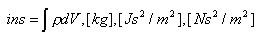Concept of the Mass

As follows from classic physics, mass is a quantitative measure of matter. For preventing logical inconsistencies it should be invariant for any movement of substance in space and time.

For such measure there corresponds only "chemical mass", definite in laboratory conditions, when the motion of matter does not make a measure of mass ambiguous. In 20-th century physics the "rest mass" approximately corresponds to such measure.

The rest-mass can be measured by two, in a strong sense nonequivalent ways:

· by usage of gravity (heavy, gravitational mass),

· by usage of elastic force (inert mass).

In both cases the process of matching with the measurement standard is indispensable.

In connection with practical equivalence of heavy and inert mass, and also technological features of process of measurement substantially there is a mixed process of definition of mass, in which one sometimes it is difficult to select the type of measured mass.

In connection with tangle reigning in physics, we will give the unambiguous definitions of mass.

Inert mass is a quantitative measure of matter, measured by definition of inertia of the given quantity of substance. The inertia of substance is determined by response of substance (its acceleration a) on effect of measuring elastic force F in absence of effect diverse (gravitational, electrical, magnetic) forces

M = F/a [kg], [Ns2/m]

As follows from the theory of measurements measuring force and acceleration, caused by it, should not influence inert properties of measured matter.

Complex Inertia and Kinds of Energy

At the analysis of motion of a substantional body with high speeds it exhibits at once two types of inertia - substantional and reactive (field). Thus, between them and their derivative on time exist the same connection, as between complex, active and reactive energies, powers and resistance in electrical engineering .

It is possible to write, that the complex inertia equals

inc = ins + i · inr

Where i – is an imaginary unit √(-1).

For complex inertia we can draw a rectangular triangle, similar volume, which we draw for complex electrical values (see fig. 1).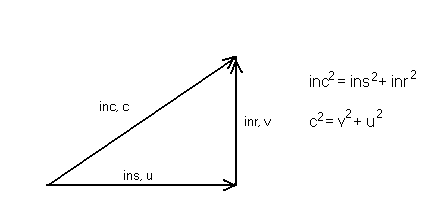Fig. 1. The triangle of inertia

Its hypotenuse maps complex (full) inertia and speed of the light. The lower leg is substantional inertia (gravitational mass) and speed of transformation of a phase Aether into corpuscular. A vertical leg is a reactive inertia - inertia of fast motion of a body and speed of this motion. In the case of the field forms of the matter, such as light, the triangle of inertia degenerates into a section of reactive inertia, when

inc = inr, ins = 0

In the case of a still body it degenerates into a section of a rest mass.

inc = ins = m, inr = 0

Therefore, considering Dirac's equation for relativistic mass and energy, it is possible to assert that it is insecurely interpreted physically. There is no relativistic mass, as quantity of substance at motion does not change. Reactive component of inertia varies only. As is clarified by present author, the change of gravitational mass during motions of the different nature is subordinate to other law .

Gravitational mass is a quantitative measure of substance, measured by definition of a strength of the influence on it of gravitational acceleration, the nature of which will be uncovered below.

According to present model of Aether both measures of mass (gravitational and inert) are caused by completely different physical processes, and therefore are in essence various. The equality of these two masss for the same body is caused only by its vanishingly small running speed in matching with speed of the light.

Reasons of a gravitation

As is discovered by present author in [2-6], the gravitation is process of a disturbance in Aether caused by substance, which changes local pressure of Aether (creates rarefaction), that causes attraction of bodies to each other.

Substantially process happens as follows. Between domains of the main, corpuscular Aether, amers of which are practically immobile and represent balance massless gyros, the phase Aether goes.

The phase Aether represents 2-dimensional (single amer layer) gas similar on properties to a saturated steam. Hypotheticalally its are "heavy" unbalanced amer-gyros. On calculations of present author effective radius of amer of a phase Aether is much more, than amers of a corpuscular Aether.

The matter, as is discovered earlier by writer, transforms amers of a phase Aether into amers of corpuscular with following speed

 Ig = 2πc2/h = 8.52 ·1050 [amer / kg s] (2)

This transformation is similar to phase transformation of devaporation. Thus practically all energy of a phase Aether amers motion passes in energy of rotary motion of corpuscular Aether amers, practically not causing temperature rise of matter. Absence of such increase is actual fact.

As a result of this transformation around of "gravitating" substance there is a pressure decrease of Aether, as is a reason of gravitation. Spherically symmetrical difference of pressure around of a gravitating body creates a gravitational field gradient. The value of pressure, as is defined by present author in  is transmitted to Aether with speed on many orders higher than speed of the light. However speed of centripetal displacement of corpuscular Aether is insignificant. It is caused by that under action of pressure, Aether being a massif of gyros, displaces not in the direction of pressure action, but in the plane, normal to the vector of pressure, according to property of precession of gyros.

The value of gravitational constant γ from Newton's formula can be received with using eq. (2)

γ = Ig c r 2 = 6.6726 ·10 -11 [m3 /kg s2]

Where c – is the speed of light,

r – is an amer radius.

Gravitons and Thermogen

As in due course "thermogen", the gravitons have played the role in construction of the ethereal theory of gravitation. We should recognize, that in connection with discovery of the nature of gravitation phenomenon , as rarefactions created by phase transformation in Aether, the concept of gravitons henceforth is redundant.

Conclusions

As a result of the study, conducted by present author, is clarified, that the phenomenon of inertia is exclusive attribute of Aether. The inertia has two components, one of which is Aether response to presence of substance (matter having rest mass), and other - for field. The complex inertia is subject to the rule of addition of known complex quantities. The relativistic masses (longitudinal and transversal) are artefacts.

The phenomenon of gravitation is a result of a gradient of pressure at phase transformation of Aether in the substance.

References

1. Khaidarov K. A. Eternal Universe. - Borovoye, 2003.
2. Khaidarov K. A. Gravitation Ether. - Borovoye, 2003.
3. Khaidarov K. A. The New Lights.. - Borovoye, 2003.
4. Khaidarov K. A. Aethereal Breathing. - Borovoye, 2003.
5. Khaidarov K. A. Aethereal Gravitation. - Almaty, 2003.
6. Khaidarov K. A. Fast Gravitation. - Borovoye, 2003.
7. Khaidarov K. A. Aethereal Atom. - Borovoye, 2004.
8. Khaidarov K. A. Aethereal Electron. - Borovoye, 2004.
9. Khaidarov K. A. Aethereal Theory of Conduction. - Borovoye, 2004.
10. Яворский Б.М., Детлаф А.А. Справочник по физике. – М., Наука, 1968.
11. Bjerknes, C. Albert Einstein: The lncorrigible Plagiarist, XTX Inc., Dowers Grove, 2002.
12. Poincare H. Lorentz Transformation and Principle of Reaction. - Ann. Neerlandes, Ser.2, 1900.
13. Бессонов Л.А. Теоретические основы электротехники. – М., Высш. школа, 1978, 528 с.
14. Физический энциклопедический словарь, Гироскопические силы, - М. СЭ, 1982.

Karim Khaidarov

Almaty, May 6, 2004.

main page   list of papers   library